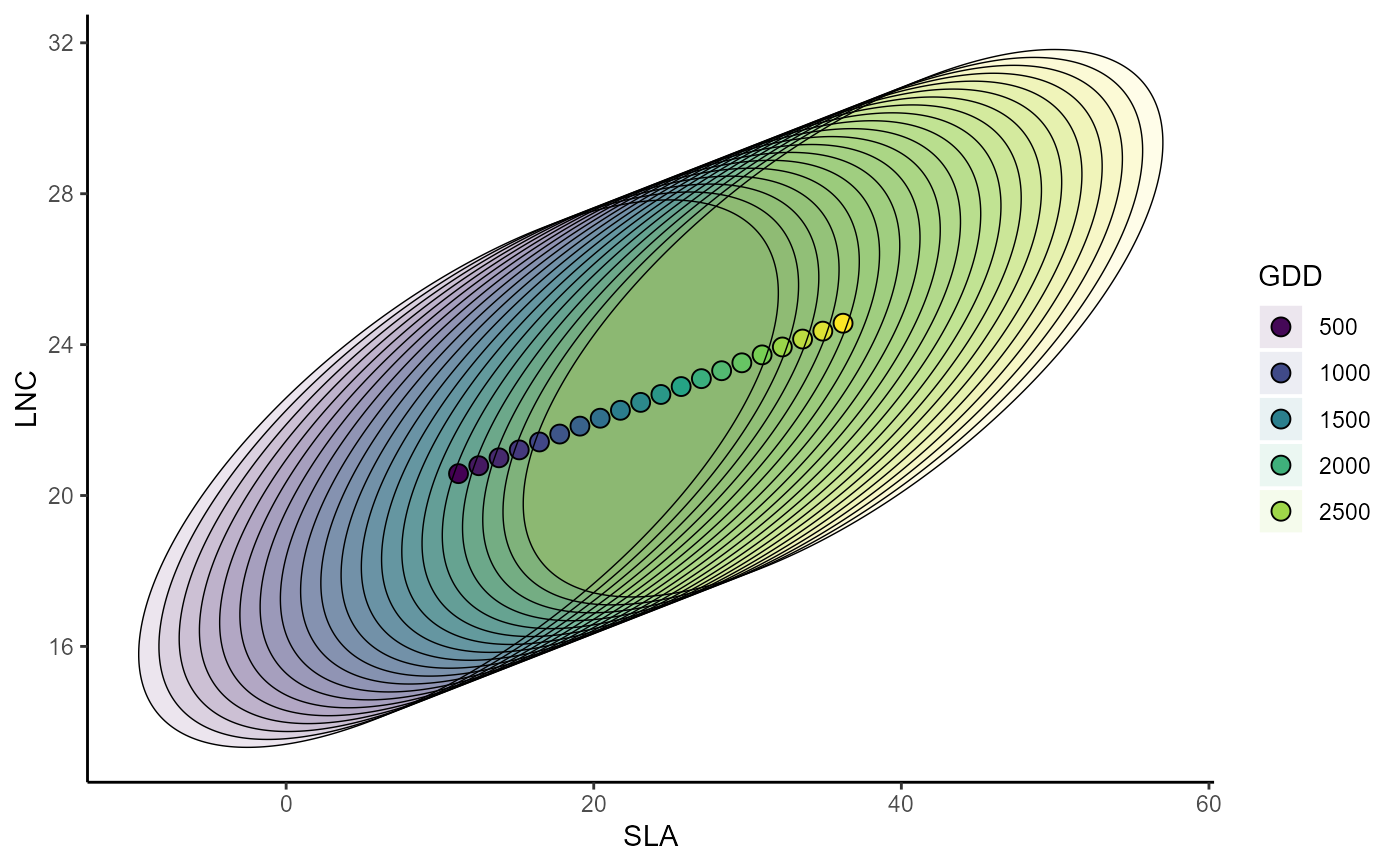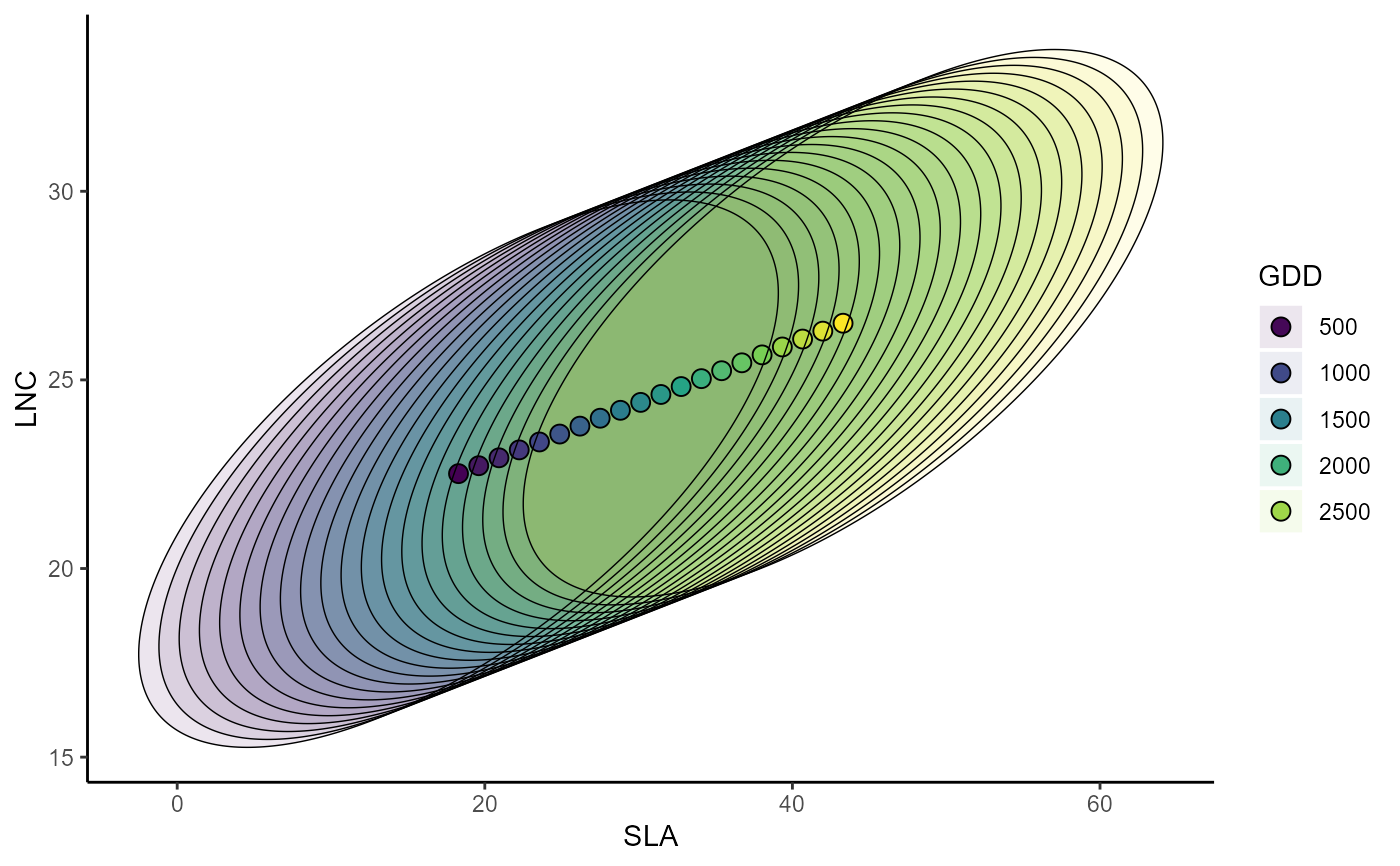Partial response curve of the pairwise most suitable community-level strategy and of the pairwise envelop of possible community-level strategy. In order to build the response curve, the function builds a dataframe where the focal variable varies along a gradient and the other (non-focal) variables are fixed to their mean (but see FixX parameter for fixing non-focal variables to user-defined values). The chosen traits are specified in indexTrait. Then uses the jtdm_predict function to compute the most suitable community-level strategy and the residual covariance matrix to build the envelop of possible CWM combinations.

ellipse_plot(
m,
indexTrait,
FullPost = FALSE,
grid.length = 20,
FixX = NULL,
confL = 0.95
)

## Arguments

m

a model fitted with jtdm_fit

The name (as specified in the column names of X) of the focal variable.

indexTrait

A vector of the two names (as specified in the column names of Y) containing the two (or more!) traits we want to compute the community level strategy of.

FullPost

If FullPost = TRUE, the function returns samples from the predictive distribution of joint probabilities. If FullPost= FALSE, joint probabilities are computed only using the posterior mean of the parameters.

grid.length

The number of points along the gradient of the focal variable. Default to 20 (which ensures a fair visualization).

FixX

Optional. A parameter to specify the value to which non-focal variables are fixed. This can be useful for example if we have some categorical variables (e.g. forest vs meadows) and we want to obtain the partial response curve for a given value of the variable. It has to be a list of the length and names of the columns of X. For example, if the columns of X are "MAT","MAP","Habitat" and we want to fix "Habitat" to 1, then FixX=list(MAT=NULL,MAP=NULL,Habitat=1.). Default to NULL.

confL

The confidence level of the confidence ellipse (i.e. of the envelop of possible community-level strategies). Default is 0.95.

## Value

Plot of the partial response curve of the pairwise most suitable community-level strategy and of the pairwise envelop of possible community-level strategy

## Examples

data(Y)
data(X)
# Short MCMC to obtain a fast example: results are unreliable !
m = jtdm_fit(Y=Y, X=X, formula=as.formula("~GDD+FDD+forest"),  sample = 1000)

# plot the pairwise SLA-LNC partial response curve along the GDD gradient